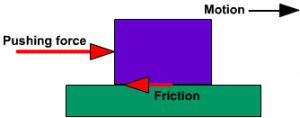Join Our WhatsApp Group!

# What is Frictional Force? – Definition & formula

Let’s start with the introduction of “What is Frictional Force”. This article, discuss about the definition of frictional force, the example of frictional force, and the formula of frictional force.

Contents

## What is Frictional Force?

The frictional force is the force exerted by a surface as an object moves across it or makes an effort to move across it. The frictional force often opposes the motion of the object. For example, if a notebook slides across the surface of the desk then the desk exerts a frictional force in the direction of its motion.Friction results from the two surfaces being pressed together closely, causing intermolecular attractive forces between the molecules of different surfaces. As such, friction depends upon the nature of the two surfaces and the degree to which they are pressed together. The maximum amount of frictional force that a surface can exert upon an object is

Ffrict = μN

Ffrict = μ x Fnorm

Where μ  is the coefficient of friction.

The kinetic friction can be sliding or rolling, and fluid friction.

Static friction> Sliding friction> Rolling friction> Fluid friction

### Static Friction

The force of friction that comes into play between two bodies before one body actually starts moving over the other is known as static friction.

### Limiting friction

The maximum force of static friction which comes into play when a body just starts moving over the surface of another body is known as limiting friction.

### Kinetic or Dynamic Friction

The force of friction that comes into play when a body is in a state of steady motion over the surface of another body is called kinetic or dynamic friction.

## Factors affecting the force of friction

Mass and Weight: As it increases, the force of friction increases too such as the force required to overcome friction (in order to pull the object forward) increases.

Nature of surface: As the roughness of the surface increases, the force of friction also increases. Therefore, the force needed to overcome friction (pull the object forward) increases.

The force of friction is not affected by the amount of surface area of an object but on the surface area in contact. Therefore, in kinetic friction, the rolling friction is less than the sliding friction.